# Lesson 20.ppt

Apr. 3, 2023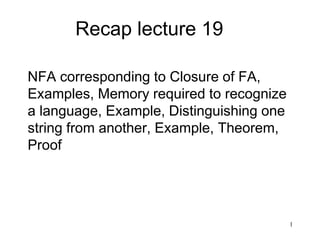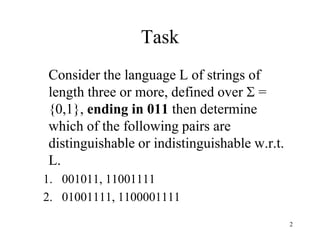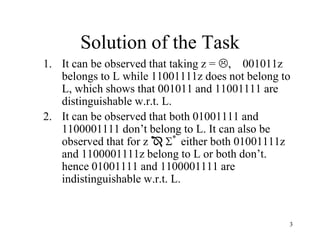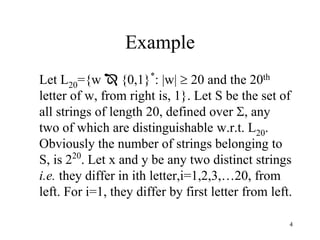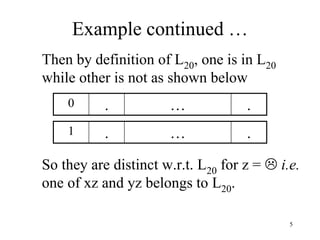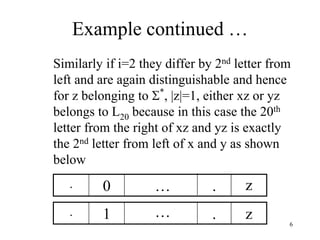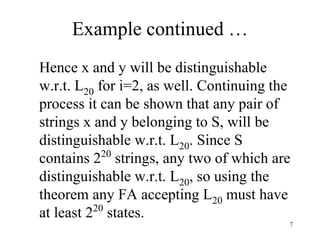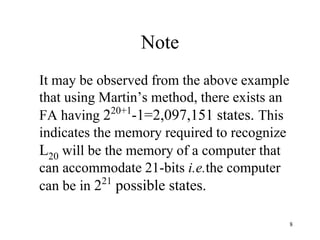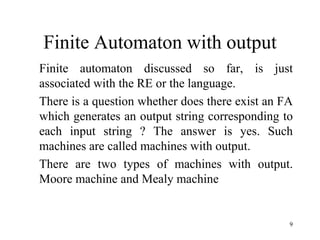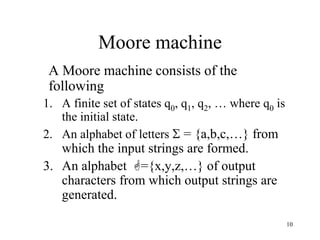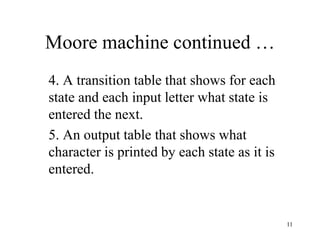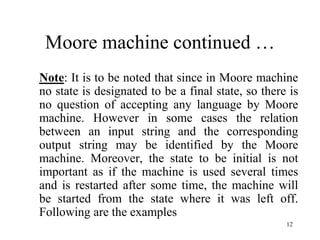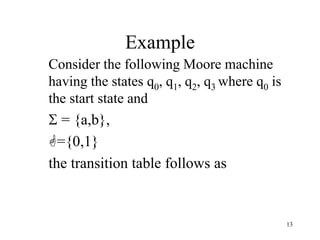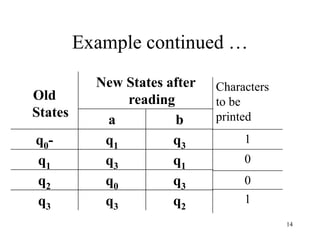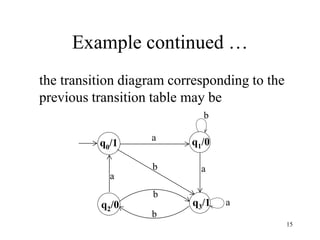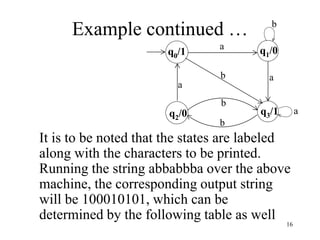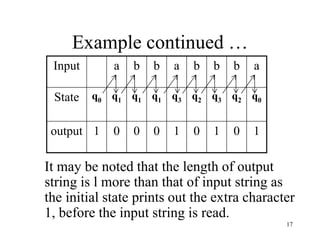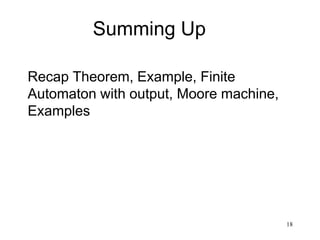1 of 18

### Lesson 20.ppt

• 1. 1 Recap lecture 19 NFA corresponding to Closure of FA, Examples, Memory required to recognize a language, Example, Distinguishing one string from another, Example, Theorem, Proof
• 2. 2 Task Consider the language L of strings of length three or more, defined over  = {0,1}, ending in 011 then determine which of the following pairs are distinguishable or indistinguishable w.r.t. L. 1. 001011, 11001111 2. 01001111, 1100001111
• 3. 3 Solution of the Task 1. It can be observed that taking z = , 001011z belongs to L while 11001111z does not belong to L, which shows that 001011 and 11001111 are distinguishable w.r.t. L. 2. It can be observed that both 01001111 and 1100001111 don’t belong to L. It can also be observed that for z  * either both 01001111z and 1100001111z belong to L or both don’t. hence 01001111 and 1100001111 are indistinguishable w.r.t. L.
• 4. 4 Example Let L20={w  {0,1}* : |w|  20 and the 20th letter of w, from right is, 1}. Let S be the set of all strings of length 20, defined over , any two of which are distinguishable w.r.t. L20. Obviously the number of strings belonging to S, is 220 . Let x and y be any two distinct strings i.e. they differ in ith letter,i=1,2,3,…20, from left. For i=1, they differ by first letter from left.
• 5. 5 Example continued … Then by definition of L20, one is in L20 while other is not as shown below So they are distinct w.r.t. L20 for z =  i.e. one of xz and yz belongs to L20. . … . 0 . … . 1
• 6. 6 Example continued … Similarly if i=2 they differ by 2nd letter from left and are again distinguishable and hence for z belonging to * , |z|=1, either xz or yz belongs to L20 because in this case the 20th letter from the right of xz and yz is exactly the 2nd letter from left of x and y as shown below . … 0 . . … 1 . z z
• 7. 7 Example continued … Hence x and y will be distinguishable w.r.t. L20 for i=2, as well. Continuing the process it can be shown that any pair of strings x and y belonging to S, will be distinguishable w.r.t. L20. Since S contains 220 strings, any two of which are distinguishable w.r.t. L20, so using the theorem any FA accepting L20 must have at least 220 states.
• 8. 8 Note It may be observed from the above example that using Martin’s method, there exists an FA having 220+1 -1=2,097,151 states. This indicates the memory required to recognize L20 will be the memory of a computer that can accommodate 21-bits i.e.the computer can be in 221 possible states.
• 9. 9 Finite Automaton with output Finite automaton discussed so far, is just associated with the RE or the language. There is a question whether does there exist an FA which generates an output string corresponding to each input string ? The answer is yes. Such machines are called machines with output. There are two types of machines with output. Moore machine and Mealy machine
• 10. 10 Moore machine A Moore machine consists of the following 1. A finite set of states q0, q1, q2, … where q0 is the initial state. 2. An alphabet of letters  = {a,b,c,…} from which the input strings are formed. 3. An alphabet ={x,y,z,…} of output characters from which output strings are generated.
• 11. 11 Moore machine continued … 4. A transition table that shows for each state and each input letter what state is entered the next. 5. An output table that shows what character is printed by each state as it is entered.
• 12. 12 Moore machine continued … Note: It is to be noted that since in Moore machine no state is designated to be a final state, so there is no question of accepting any language by Moore machine. However in some cases the relation between an input string and the corresponding output string may be identified by the Moore machine. Moreover, the state to be initial is not important as if the machine is used several times and is restarted after some time, the machine will be started from the state where it was left off. Following are the examples
• 13. 13 Example Consider the following Moore machine having the states q0, q1, q2, q3 where q0 is the start state and  = {a,b}, ={0,1} the transition table follows as
• 14. 14 Example continued … Old States New States after reading a b q0- q1 q3 q1 q3 q1 q2 q0 q3 q3 q3 q2 1 0 0 1 Characters to be printed
• 15. 15 Example continued … the transition diagram corresponding to the previous transition table may be a b b a q0/1 a b q1/0 q2/0 q3/1 a b
• 16. 16 Example continued … It is to be noted that the states are labeled along with the characters to be printed. Running the string abbabbba over the above machine, the corresponding output string will be 100010101, which can be determined by the following table as well a b b a q0/1 a b q1/0 q2/0 q3/1 a b
• 17. 17 Example continued … 1 0 1 0 1 0 0 0 1 output q0 q2 q3 q2 q3 q1 q1 q1 q0 State a b b b a b b a Input It may be noted that the length of output string is l more than that of input string as the initial state prints out the extra character 1, before the input string is read.
• 18. 18 Summing Up Recap Theorem, Example, Finite Automaton with output, Moore machine, Examples